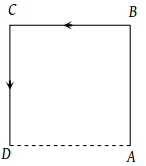Read the assertion and reason carefully to mark the correct option out of the options given below:

(1) If both assertion and reason are true and the reason is the correct explanation of the assertion.

(2) If both assertion and reason are true but reason is not the correct explanation of the assertion.

(3) If assertion is true but reason is false.

(4) If the assertion and reason both are false.

(5) If assertion is false but reason is true.

Assertion : Displacement of a body is vector sum of the area under velocity-time graph.

Reason : Displacement is a vector quantity.

Concept Questions :-

Graphs
High Yielding Test Series + Question Bank - NEET 2020

Difficulty Level:

Read the assertion and reason carefully to mark the correct option out of the options given below:

(1) If both assertion and reason are true and the reason is the correct explanation of the assertion.

(2) If both assertion and reason are true but reason is not the correct explanation of the assertion.

(3) If assertion is true but reason is false.

(4) If the assertion and reason both are false.

(5) If assertion is false but reason is true.

Assertion : The average speed of an object may be equal to arithmetic mean of individual speed.

Reason : Average speed is equal to total distance travelled per total time taken.

Concept Questions :-

Average speed and average velocity
High Yielding Test Series + Question Bank - NEET 2020

Difficulty Level:

Read the assertion and reason carefully to mark the correct option out of the options given below:

(1) If both assertion and reason are true and the reason is the correct explanation of the assertion.

(2) If both assertion and reason are true but reason is not the correct explanation of the assertion.

(3) If assertion is true but reason is false.

(4) If the assertion and reason both are false.

(5) If assertion is false but reason is true.

Assertion : The average and instantaneous velocities have same value in a uniform motion.

Reason : In uniform motion, the velocity of an object increases uniformly.

Concept Questions :-

Average speed and average velocity
High Yielding Test Series + Question Bank - NEET 2020

Difficulty Level:

A car travels a distance S on a straight road in two hours and then returns to the starting point in the next three hours. Its average velocity is

(1) S/5

(2) 2S/5

(3) S/2 + S/3

(4) None of the above

Concept Questions :-

Average speed and average velocity
High Yielding Test Series + Question Bank - NEET 2020

Difficulty Level:

A particle moves along the sides AB, BC, CD of a square of side 25 m with a velocity of 15 ms-1. Its average velocity is(1) 15 ms-1

(2) 10 ms-1

(3) 7.5 ms-1

(4) 5 ms-1

Concept Questions :-

Average speed and average velocity
High Yielding Test Series + Question Bank - NEET 2020

Difficulty Level:

A body has speed V, 2V and 3V in first 1/3 of distance S, second 1/3 of S and third 1/3 of S respectively. Its average speed will be

(1) V

(2) 2V

(3) $\frac{18}{11}V$

(4) $\frac{11}{18}V$

Concept Questions :-

Average speed and average velocity
High Yielding Test Series + Question Bank - NEET 2020

Difficulty Level:

If the body covers one-third distance at speed v1, next one third at speed v2 and last one third at speed v3, then average speed will be

(1) $\frac{{v}_{1}\text{\hspace{0.17em}}{v}_{2}+{v}_{2}\text{\hspace{0.17em}}{v}_{3}+{v}_{3}\text{\hspace{0.17em}}{v}_{1}}{{v}_{1}+{v}_{2}+{v}_{3}}$

(2) $\frac{{v}_{1}+{v}_{2}+{v}_{3}}{3}$

(3) $\frac{{v}_{1}{v}_{2}{v}_{3}}{{v}_{1}\text{\hspace{0.17em}}{v}_{2}+{v}_{2}\text{\hspace{0.17em}}{v}_{3}+{v}_{3}\text{\hspace{0.17em}}{v}_{1}}$

(4) $\frac{3{v}_{1}{v}_{2}{v}_{3}}{{v}_{1}\text{\hspace{0.17em}}{v}_{2}+{v}_{2}\text{\hspace{0.17em}}{v}_{3}+{v}_{3}\text{\hspace{0.17em}}{v}_{1}}$

Concept Questions :-

Average speed and average velocity
High Yielding Test Series + Question Bank - NEET 2020

Difficulty Level:

The displacement of the particle varies with time according to the relation $x=\frac{k}{b}\text{\hspace{0.17em}}\left[1-{e}^{-bt}\right]$. Then the velocity of the particle is

(1) $k\text{\hspace{0.17em}}\left({e}^{-bt}\right)$

(2) $\frac{k}{{b}^{2}{e}^{-bt}}$

(3) $k\text{\hspace{0.17em}}b\text{\hspace{0.17em}}{e}^{-bt}$

(4) None of these

Concept Questions :-

Instantaneous speed and instantaneous velocity
High Yielding Test Series + Question Bank - NEET 2020

Difficulty Level:

The acceleration of a particle starting from rest, varies with time according to the relation A = – 2 sinω t. The displacement of this particle at a time t will be

(1) $-\frac{1}{2}\text{\hspace{0.17em}}\left(a{\omega }^{2}\mathrm{sin}\omega \text{\hspace{0.17em}}t\right)\text{\hspace{0.17em}}{t}^{2}$

(2) $a\omega \text{\hspace{0.17em}}\mathrm{sin}\omega \text{\hspace{0.17em}}t$

(3) $a\omega \text{\hspace{0.17em}}\mathrm{cos}\omega \text{\hspace{0.17em}}t$

(4) $a\text{\hspace{0.17em}}\mathrm{sin}\omega \text{\hspace{0.17em}}t$

Concept Questions :-

Non-uniform acceleration
High Yielding Test Series + Question Bank - NEET 2020

Difficulty Level:

If the velocity of a particle is (10 + 2t2) m/s, then the average acceleration of the particle between 2s and 5s is

(1) 2 m/s2

(2) 4 m/s2

(3) 12 m/s2

(4) 14 m/s2

Concept Questions :-

Non-uniform acceleration